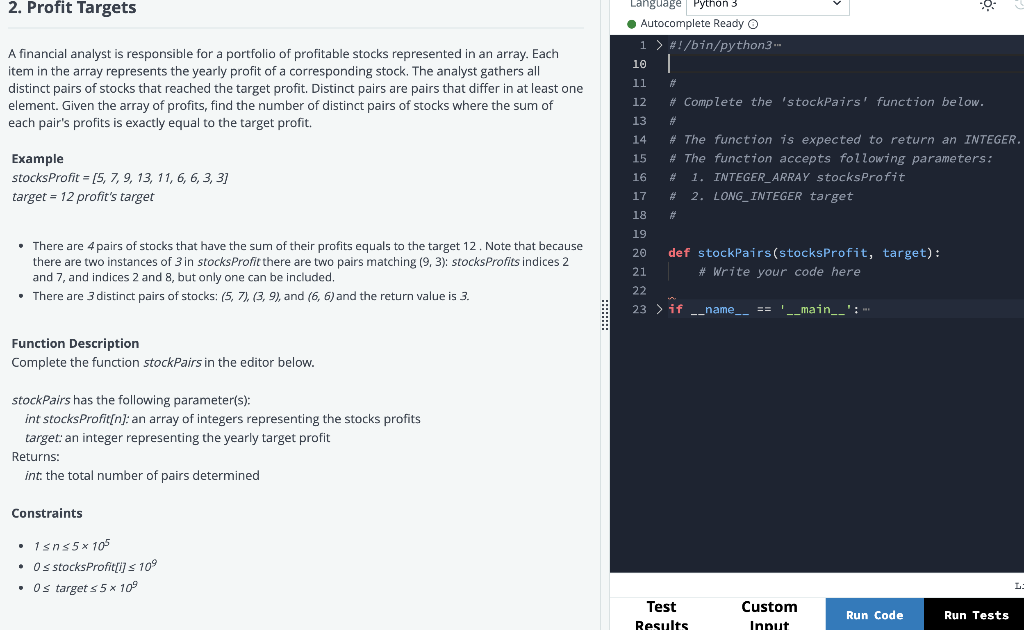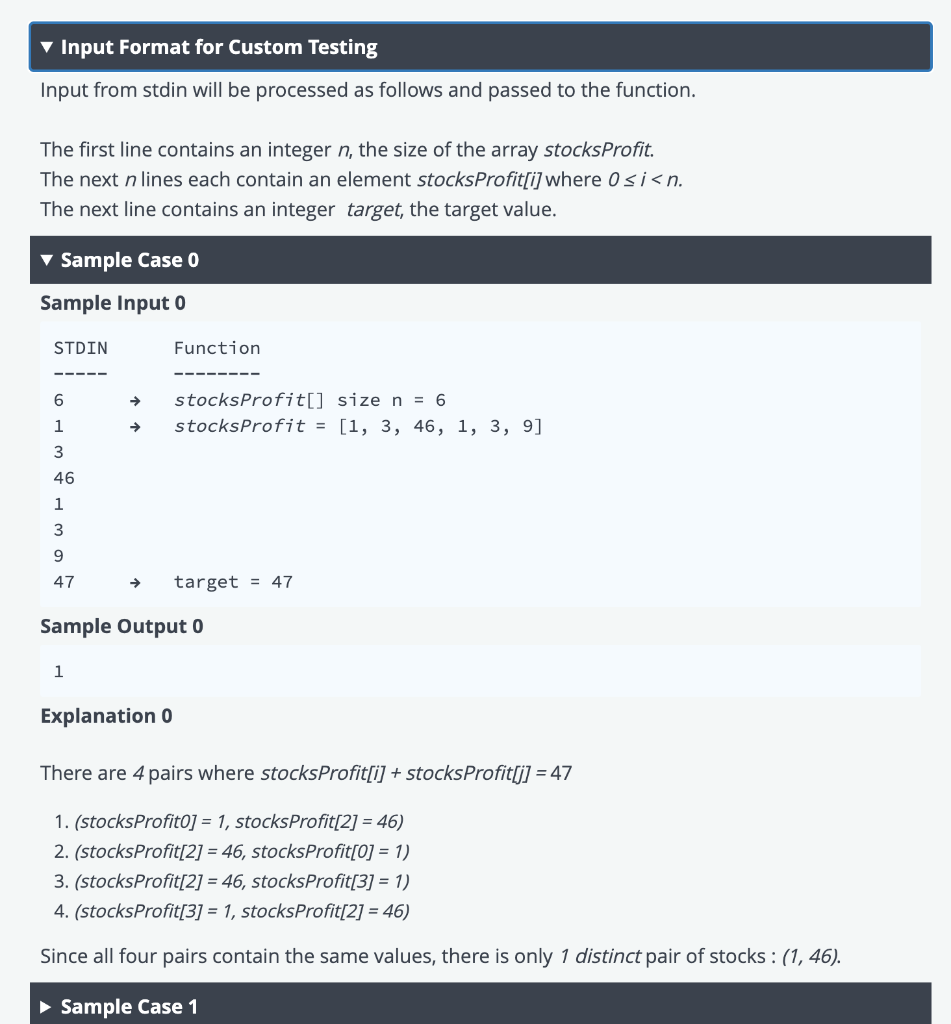# 線上程式面試的進行方式Profit Targets A Financial Analyst Is Responsible For A Portfolio Of Profitable Stocks Represented In An Array2022-01-07 | 2 min.

## 問題理解

A financial analyst is responsible for a portfolio of profitable stocks represented in an array. Each item in the array represents the yearly profit of a corresponding stock. The analyst gathers all distinct pairs of stocks that reached the target profit. Distinct pairs are pairs that differ in at least one element. Given the array of profits, find the number of distinct pairs of stocks where the sum of each pair’s profits is exactly equal to the target profit.## 解題技巧與步驟

stocksProfit = [5, 7, 9, 13, 11, 6, 6, 3, 3]

target = 12 profit’s target

There are 4 pairs of stocks that have the sum of their profits equals to the target 12 . Note that because there are two instances of 3 in stocksProfit there are two pairs matching (9, 3): stocksProfits indices 2 and 7, and indices 2 and 8, but only one can be included.
There are 3 distinct pairs of stocks: (5, 7), (3, 9), and (6, 6) and the return value is 3.

• 是否有多跑的迴圈、遞迴
• 資料儲存型態
• 判斷條件